SSC  >  Test: General Intelligence & Reasoning - 1

# Test: General Intelligence & Reasoning - 1

Test Description

## 50 Questions MCQ Test Mock Test Series for SSC JE Mechanical Engineering | Test: General Intelligence & Reasoning - 1

Test: General Intelligence & Reasoning - 1 for SSC 2023 is part of Mock Test Series for SSC JE Mechanical Engineering preparation. The Test: General Intelligence & Reasoning - 1 questions and answers have been prepared according to the SSC exam syllabus.The Test: General Intelligence & Reasoning - 1 MCQs are made for SSC 2023 Exam. Find important definitions, questions, notes, meanings, examples, exercises, MCQs and online tests for Test: General Intelligence & Reasoning - 1 below.
Solutions of Test: General Intelligence & Reasoning - 1 questions in English are available as part of our Mock Test Series for SSC JE Mechanical Engineering for SSC & Test: General Intelligence & Reasoning - 1 solutions in Hindi for Mock Test Series for SSC JE Mechanical Engineering course. Download more important topics, notes, lectures and mock test series for SSC Exam by signing up for free. Attempt Test: General Intelligence & Reasoning - 1 | 50 questions in 50 minutes | Mock test for SSC preparation | Free important questions MCQ to study Mock Test Series for SSC JE Mechanical Engineering for SSC Exam | Download free PDF with solutions
 1 Crore+ students have signed up on EduRev. Have you?
Test: General Intelligence & Reasoning - 1 - Question 1

### In the following question, select the related word from the given alternatives. Scissors : Cloth :: Axe : ?

Detailed Solution for Test: General Intelligence & Reasoning - 1 - Question 1

As scissors is used for cutting the clothes,

Similarly,

Axe is used for cutting wood.

Hence, the correct answer is wood.

Test: General Intelligence & Reasoning - 1 - Question 2

### In the following question, select the related word from the given alternatives. Fan : Blades : : ? : ?

Detailed Solution for Test: General Intelligence & Reasoning - 1 - Question 2

Fan contains blades as its major component. Similarly, Book contains chapters as its major component.

Hence, Book : Chapter is the correct ans.

Test: General Intelligence & Reasoning - 1 - Question 3

### In question, select the related word from the given alternatives. Elated : Despondent :: Enlightened : ____

Detailed Solution for Test: General Intelligence & Reasoning - 1 - Question 3

Elated means happy which is the opposite of despondent that means discourage. Similarly, enlightened is the opposite of ignorant.

Test: General Intelligence & Reasoning - 1 - Question 4

In question below, select the related letters from the given alternative.

BYCX :DWEV :: FUGT : ?

Test: General Intelligence & Reasoning - 1 - Question 5

Select the related letters from the given alternatives.

DBCE: QOPR :: JLKI :?

Test: General Intelligence & Reasoning - 1 - Question 6

Directions: In the following question, select the odd letters from the given alternatives.

ACEG : IKMO :: QSUW : ?

Test: General Intelligence & Reasoning - 1 - Question 7

In question select the related numbers from the given alternatives.

76 : 01 :: 42 : ?

Detailed Solution for Test: General Intelligence & Reasoning - 1 - Question 7

The difference between the first two digits gives the second number.

For example, 7 – 6 = 1.

Similarly, 4 – 2 = 2.

Therefore, the final pairing will be 76 : 01 ∷ 42 : 2.

Test: General Intelligence & Reasoning - 1 - Question 8

Select the related numbers from the given alternatives :
35 : 48 :: 110 : ?

Detailed Solution for Test: General Intelligence & Reasoning - 1 - Question 8

In 35 : 48

7 × 5 = 35

8 × 6 = 48

Similarly;

11 × 10 = 110

12 × 11 = 132

Hence, 132 is correct option.

Test: General Intelligence & Reasoning - 1 - Question 9

Select the related number from the given alternatives.

36 : 25 : : 100 : ?

Detailed Solution for Test: General Intelligence & Reasoning - 1 - Question 9

In 36 : 25

⇒ 62 : 52

⇒ 62 : (6 – 1)2

Similarly,

⇒ 100 : ?

⇒ 102 : (10 – 1)2

⇒ 102 : 92

100 : 81

Hence, “81” is required number.

Test: General Intelligence & Reasoning - 1 - Question 10

Find the odd word from the given alternatives.

Detailed Solution for Test: General Intelligence & Reasoning - 1 - Question 10

Square, Rectangle and Circle are 2-dimensional figures while Sphere is a 3-dimensional figure.

Test: General Intelligence & Reasoning - 1 - Question 11

Directions: Select the one which is different from the other three responses.

Detailed Solution for Test: General Intelligence & Reasoning - 1 - Question 11

Ear, Eye and Nose are the sensory organs but neck is not.

Hence Neck is different from other responses.

Test: General Intelligence & Reasoning - 1 - Question 12

In the following question, select the related word pair from the given alternatives.

Rabbit : Animal : : ? : ?

Detailed Solution for Test: General Intelligence & Reasoning - 1 - Question 12

Just as Rabbit is an animals, Pentagon is a Figure.

Test: General Intelligence & Reasoning - 1 - Question 13

Select the odd letters from the given alternatives.

Detailed Solution for Test: General Intelligence & Reasoning - 1 - Question 13

OP, IJ, DE are consecutive letter in sequence but VU are consecutive letter but not in sequence.

Therefore, VU is odd one out.

Test: General Intelligence & Reasoning - 1 - Question 14

Direction: Select the one which is different from the other three responses :

Detailed Solution for Test: General Intelligence & Reasoning - 1 - Question 14

ABCD is continuous series while in other series there are having some missing letters in between.

Test: General Intelligence & Reasoning - 1 - Question 15

Directions: Find the odd letters from the given alternatives.

Detailed Solution for Test: General Intelligence & Reasoning - 1 - Question 15

M + 1 = N, D + 1 = E

H + 1 = I, X + 1 = Y

L + 1 = M, V + 1 = W

R – 1 = Q, C – 1 = B

The odd one out is RQCB.

Test: General Intelligence & Reasoning - 1 - Question 16

Direction: In the question given find the odd number from the given alternatives.

Detailed Solution for Test: General Intelligence & Reasoning - 1 - Question 16

Here, 976, 778 and 895 are consecutive number whereas 997 is a prime number.

Therefore, “997” is odd one among given alternatives.

Test: General Intelligence & Reasoning - 1 - Question 17

Find the odd number pair from the given alternatives.

Detailed Solution for Test: General Intelligence & Reasoning - 1 - Question 17

The digits of the given pairs of numbers are in reverse order of each other.

Hence odd should be 96-19 because instead of 19 it should be 69.

Test: General Intelligence & Reasoning - 1 - Question 18

Find the odd number pair from the given alternatives.

Detailed Solution for Test: General Intelligence & Reasoning - 1 - Question 18

Here the pattern is : Number - Number × 2.

36 ­ 72 → 36 - 36 × 2 = 72

17 ­ 34 → 17 - 17 × 2 = 34

28 ­ 49 → 28 - 28 × 2 = 56

24 ­ 48 → 24 - 24 × 2 = 48

Thus 28 - 49, is the odd one out.

Test: General Intelligence & Reasoning - 1 - Question 19

Which of the following terms follows the trend of the given list?

YXXXXXX, YYXXXXX, YYYXXXX, YYYYXXX, YYYYYXX, _______________.

Detailed Solution for Test: General Intelligence & Reasoning - 1 - Question 19

The pattern here is the letter ‘X’ which is to the immediate right of letter ‘Y’ is converted to ‘Y’ in every step and hence increasing the number of letter ‘Y’ in every step i.e.

YXXXXXX, YYXXXXX, YYYXXXX, YYYYXXX, YYYYYXX, YYYYYYX.

Hence, YYYYYYX is the correct alternative.

Test: General Intelligence & Reasoning - 1 - Question 20

A series is given with one term missing. Select the correct alternative from the given ones that will complete the series.

YZ, VW, ST, ?, MN

Test: General Intelligence & Reasoning - 1 - Question 21

A series is given with one term missing. Select the correct alternative from the given ones that will complete the series.

POT, OMS, NKR, MIQ, ?

Detailed Solution for Test: General Intelligence & Reasoning - 1 - Question 21

P – 1 = O, O – 1 = N, N- 1 – M, M- 1 = L

O – 2 = M, M- 2 = K, K – 2 = I, I – 2 = G

T – 1 = S, S- 1 = R, R- 1 = Q, Q – 1= P

Hence LGP is the correct answer.

Test: General Intelligence & Reasoning - 1 - Question 22

A series is given with one term missing. Select the correct alternative from the given ones that will complete the series.

RMT, PKR, NIP, ?, JEL

Test: General Intelligence & Reasoning - 1 - Question 23

In the following question, select the missing number from the given series.

18, 14, 10, 6, ?

Detailed Solution for Test: General Intelligence & Reasoning - 1 - Question 23

The relation between the numbers is as follows,

18 - 4 = 14

14 - 4 = 10

10 - 4 = 6

Hence, the next number is 6 - 4 = 2.

Test: General Intelligence & Reasoning - 1 - Question 24

A series is given, with one number missing. Choose the correct alternative from the given ones that will complete the series.

-4, ?, 1, 3.5, 6, 8.5

Detailed Solution for Test: General Intelligence & Reasoning - 1 - Question 24

The pattern followed is: (+ 2.5) is added in each number.

→ -4 + 2.5 = -1.5

→ -1.5 + 2.5 = 1

→ 1 + 2.5 = 3.5

→ 3.5 + 2.5 = 6

→ 6 + 2.5 = 8.5

→ 8.5

Hence, the missing number is 8.5 in the given series.

Test: General Intelligence & Reasoning - 1 - Question 25

Replace the “?” in the following series.

7, 7, 14, 42, ?, 840

Detailed Solution for Test: General Intelligence & Reasoning - 1 - Question 25

7

7 x 1= 7

7 x 2 x 1= 14

7 x 3 x 2 x 1 = 42

7 x 4 x 3 x 2 x 1 = 168

7 x 5 x 4 x 3 x 2 x 1 = 840

Hence missing number is 168.

Test: General Intelligence & Reasoning - 1 - Question 26

In a certain code language, 462 means 'lion is wild', 543 means 'cat is hungry', 165 means 'wild and hungry'. Find the code for 'and'.

Test: General Intelligence & Reasoning - 1 - Question 27

If ANTIQUES is coded as DQWLTXHV, then how will FOX be coded as?

Detailed Solution for Test: General Intelligence & Reasoning - 1 - Question 27

ANTIQUES is coded as DQWLTXHV

⇒ A + 3 = D

⇒ N + 3 = Q

⇒ T + 3 = W

⇒ I + 3 = L

⇒ Q + 3 = T

⇒ U + 3 = X

⇒ E + 3 = H

⇒ S + 3 = V

Similarly,

⇒ F + 3 = I

⇒ O + 3 = R

⇒ X + 3 = A

Hence, in this language “FOX” will be coded as “ IRA”.

Test: General Intelligence & Reasoning - 1 - Question 28

Ram walked 20 m towards the north. Then he turned right and walks 30 m. Then he turns right and walks 35 m. Then he turns left and walks 15 m. Finally he turns left and walks 15 m. In which direction and how many metres is he from the starting position?

Test: General Intelligence & Reasoning - 1 - Question 29

Pointing to a lady in the photograph, Sakshi said, ‘Her son’s father is the son-in-law of my mother”. How is the lady related to sakshi?

Test: General Intelligence & Reasoning - 1 - Question 30

Shyama, a woman, says that father of Rajiv’s father is my father. How Shyama is related to Rajiv?

Detailed Solution for Test: General Intelligence & Reasoning - 1 - Question 30

Shyama, a woman, says that father of Rajiv’s father is my father.

Now, father of Rajiv’s father is Rajiv’s grandfather.

That means Shyama’s father is Rajiv’s grandfather.

Thus Syama is Rajiv’s aunt.

Test: General Intelligence & Reasoning - 1 - Question 31

If ‘A + B’ stands for ‘A is the father of B’, ‘A – B’ stands for ‘A is the brother of B’, ‘A × B’ stands for ‘A is the wife of B’ and ‘A ÷ B’ stands for ‘A is the sister of B’ then ‘P + Q ÷ R’ means ?

Detailed Solution for Test: General Intelligence & Reasoning - 1 - Question 31

Given conditions is: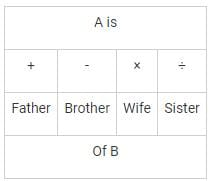Now, P + Q ÷ R means

P is father of Q and Q is sister of R.

This means P is father of R is true & other options are irrelevant as Gender of R can’t be determined with the given data.

Test: General Intelligence & Reasoning - 1 - Question 32

Arrange the given words in the sequence in which they occur in the dictionary.

1. Counter

2. Crop

3. Create

4. Carnation

5. Creator

Detailed Solution for Test: General Intelligence & Reasoning - 1 - Question 32

4. Carnation

1. Counter

3. Create

5. Creator

2. Crop

Hence, correct dictionary order is 41352.

Test: General Intelligence & Reasoning - 1 - Question 33

Arrange the following in dictionary order. Then which will be the second word?

1) Specific

2) Spectacle

3) Specified

4) Spectacular

Detailed Solution for Test: General Intelligence & Reasoning - 1 - Question 33

In dictionary order, words are put in alphabetically according to the first letter in which they differ.

Thus, the order will be:

Specifi(1), Specified (3), Spectacle (2), Spectacular (4)

Test: General Intelligence & Reasoning - 1 - Question 34

Directions: In the following questions, which one of the following words cannot be formed form the letters of the given word (using each letter once)?

VACCINATION

Detailed Solution for Test: General Intelligence & Reasoning - 1 - Question 34

1. VACATION = can be formed as VACCINATION

2. NATION = can be formed as VACCINATION

3. CIVIC = can be formed as VACCINATION

4. NICE = cannot be formed as E is not present in VACCINATION

Test: General Intelligence & Reasoning - 1 - Question 35

In the following question below are given some statements followed by some conclusions. Taking the given statements to be true even if they seem to be at variance from commonly known facts, read all the conclusions and then decide which of the given conclusion logically follows the given statements.

Statements:

I. Some pen are black.

II. Some pen are blue.

III. No black is red.

Conclusions:

I. No pen is red.

II. Some blue are black.

III. Some black are pens.

Test: General Intelligence & Reasoning - 1 - Question 36

Given below are two statements followed by two conclusions. Assuming these statements to be true, decide which one logically follow.

Statements:

I. All film stars are playback singers

II. All film directors are film stars.

Conclusions:

I. All film directors are playback singers

II. Some film star are film directors

Test: General Intelligence & Reasoning - 1 - Question 37

Select the missing number in the pattern from the given responses.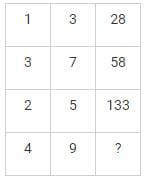Detailed Solution for Test: General Intelligence & Reasoning - 1 - Question 37

1st row = 13 + 3= 1 + 27 = 28

2nd Row = 32 + 72 = 9 + 49 = 58

3rd row = 23 + 53 = 8 + 125 = 133

4th row = 42 + 92 = 16 + 81 = 97

Therefore 97 is odd one among them.

Test: General Intelligence & Reasoning - 1 - Question 38

Some friends went out for a walk. Divya walked before farhan. Sumit was walking behind mona and ahead of farhan. Who walked last?

Detailed Solution for Test: General Intelligence & Reasoning - 1 - Question 38

According to the question, Divya walked before farhan

Divya ← Farhan

Also, Sumit was walking behind mona and ahead of farhan

Mona ← Sumit ← Farhaan

Combining both the situation we get;

Mona ← Sumit ← Divya ← Farhaan

Hence, Farhaan is walking at last

Test: General Intelligence & Reasoning - 1 - Question 39

If ‘ + ’ means ‘multiplication, ‘ - ’ means ‘division’, ‘×’ means ‘subtraction’ and ‘÷’ means ‘addition’, then 9 + 8 ÷ 8 – 4 × 9 is

Detailed Solution for Test: General Intelligence & Reasoning - 1 - Question 39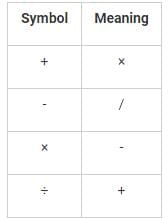We use BODMAS to calculate the following expression.

9 + 8 ÷ 8 – 4 × 9 = 9 × 8 + 8/4 – 9 = 9 × 8 + 2 – 9 = 72 + 2 – 9 = 74 – 9 = 65.

Therefore, 65 is the correct answer.

Test: General Intelligence & Reasoning - 1 - Question 40

If 18 × 12 = 206 and 19 × 22 = 408, then

23 × 36 = ?

Detailed Solution for Test: General Intelligence & Reasoning - 1 - Question 40

The pattern followed here is:

In 18 × 12 = 206 → 18 × 12 = 216, 216 - 10 = 206

In 19 × 22 = 408 → 19 × 22 = 418, 418 - 10 = 408

Hence, In 23 x 36 = ?

23 × 36 = 828, 828 - 10 = 818

Test: General Intelligence & Reasoning - 1 - Question 41

Which one of the following figures represents the relationship among Shirts, Bed sheets and Towels?

Test: General Intelligence & Reasoning - 1 - Question 42

Study the following figure and answer the question given below.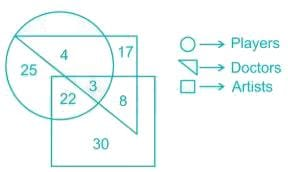How many artists are players?

Detailed Solution for Test: General Intelligence & Reasoning - 1 - Question 42

Square represents artists.

Triangle represents doctors.

Circle represents players.

Therefore, the number of artists who are players = 22 + 3 = 25.

Test: General Intelligence & Reasoning - 1 - Question 43

Directions: In question, which among the answer figures can be constructed from the parts given in question figure?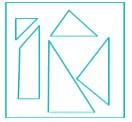Detailed Solution for Test: General Intelligence & Reasoning - 1 - Question 43

Option (C) and (D) are incorrect because they do not contain all the parts of the question figure. Option (A) triangles are not in proportion as in question figure. Hence answer is (B).

Test: General Intelligence & Reasoning - 1 - Question 44

If a mirror is placed on the line AB, then which of the answer figures is the water image of the given figure?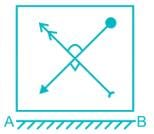Test: General Intelligence & Reasoning - 1 - Question 45

From the given figures, select the one in which the question figure is hidden/embedded.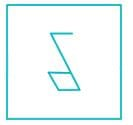Test: General Intelligence & Reasoning - 1 - Question 46

Identify the figure that completes the pattern.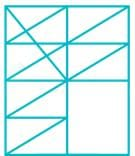Test: General Intelligence & Reasoning - 1 - Question 47

Which answer figure will complete the pattern in the question figure?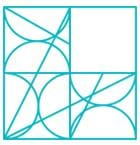Test: General Intelligence & Reasoning - 1 - Question 48

Which of the following cube in the answer figure cannot be made based on the unfolded cube in the question figure?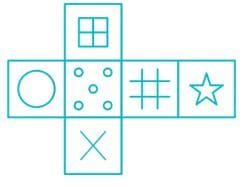Test: General Intelligence & Reasoning - 1 - Question 49

How many quadrilaterals can be found out from the following figure?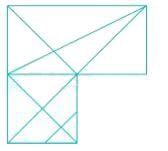Test: General Intelligence & Reasoning - 1 - Question 50

In the question, a word is represented by only one set of numbers as given in any one of the alternatives. The sets of numbers given in the alternatives are represented by two classes of alphabets as in two matrices given below. The columns and rows of Matrix I are numbered from 0 to 4 and that of Matrix II are numbered from 5 to 9. A letter from these matrices can be represented first by its row and next by its column, e.g., 'R' can be represented by 55, 67 , etc., and 'E' can be represented by 11, 22, etc.

Similarly, you have to identify the set for the word 'COUNT'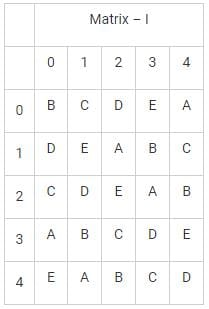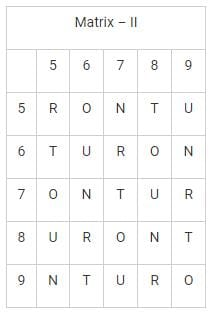Detailed Solution for Test: General Intelligence & Reasoning - 1 - Question 50

Let’s check each option

1. 20, 76, 88, 59, 75 represents CNNUO. Hence, definitely not true.

2. 20, 68, 66, 76, 79 represents COUNR. Hence, definitely not true.

3. 32, 56, 66, 88, 89 represents COUNT. Hence, true.

4. 75, 12, 89, 98, 42 represents OATRB. Hence, definitely not true.

So 32, 56, 66, 88, 89 is the correct answer.

## Mock Test Series for SSC JE Mechanical Engineering

3 videos|1 docs|55 tests
Information about Test: General Intelligence & Reasoning - 1 Page
In this test you can find the Exam questions for Test: General Intelligence & Reasoning - 1 solved & explained in the simplest way possible. Besides giving Questions and answers for Test: General Intelligence & Reasoning - 1 , EduRev gives you an ample number of Online tests for practice

## Mock Test Series for SSC JE Mechanical Engineering

3 videos|1 docs|55 tests(Scan QR code)